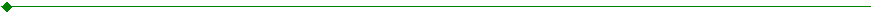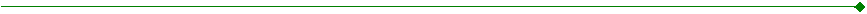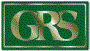June 30, 2002
EXPLANATION OF RISK/REWARD GRAPHS
 The crossing lines represent the 5-year return (horizontal line) and 5-year standard deviation or volatility or risk (vertical line) of the index against which the Fund is being measured. Each point represents the Fund's 5-year return (vertically) and standard deviation or volatility (horizontally), relative to the index.  If a point is in the southwest quadrant, for example, the 5-year return of the Fund has been less than (below) the index line, and the 5-year standard deviation (volatility) has also been less than (to the left of) the index line. There are four points, one for each of the last four quarters.  The earliest one is the smallest and the quarter just ended being the largest.  Each point shows the 5-year relative position of the Fund versus the index for that quarter.  The movement of the points shows the trend, or direction, over time.  As noted in the graph, the best place to be is the northwest quadrant (less risk and a higher return); the worst place to be is the southeast quadrant (more risk and a lower return).ASSET CONSULTING GROUP
a division of Gabriel, Roeder, Smith, & Co.